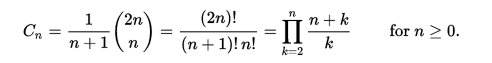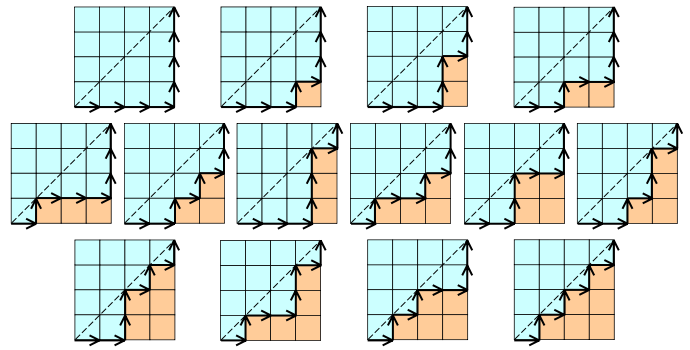# Catalan Number Algorithm Basic Information

suggest change

Catalan numbers algorithm is Dynamic Programming algorithm.

In combinatorial mathematics, the Catalan numbers form a sequence of natural numbers that occur in various counting problems, often involving recursively-defined objects. The Catalan numbers on nonnegative integers n are a set of numbers that arise in tree enumeration problems of the type, ‘In how many ways can a regular n-gon be divided into n-2 triangles if different orientations are counted separately?’

Application of Catalan Number Algorithm:

1. The number of ways to stack coins on a bottom row that consists of n consecutive coins in a plane, such that no coins are allowed to be put on the two sides of the bottom coins and every additional coin must be above two other coins, is the nth Catalan number.
2. The number of ways to group a string of n pairs of parentheses, such that each open parenthesis has a matching closed parenthesis, is the nth Catalan number.
3. The number of ways to cut an n+2-sided convex polygon in a plane into triangles by connecting vertices with straight, non-intersecting lines is the nth Catalan number. This is the application in which Euler was interested.

Using zero-based numbering, the nth Catalan number is given directly in terms of binomial coefficients by the following equation.Example of Catalan Number:

Here value of n = 4.(Best Example - From Wikipedia)Auxiliary Space: `O(n)`

Time Complexity: `O(n^2)`